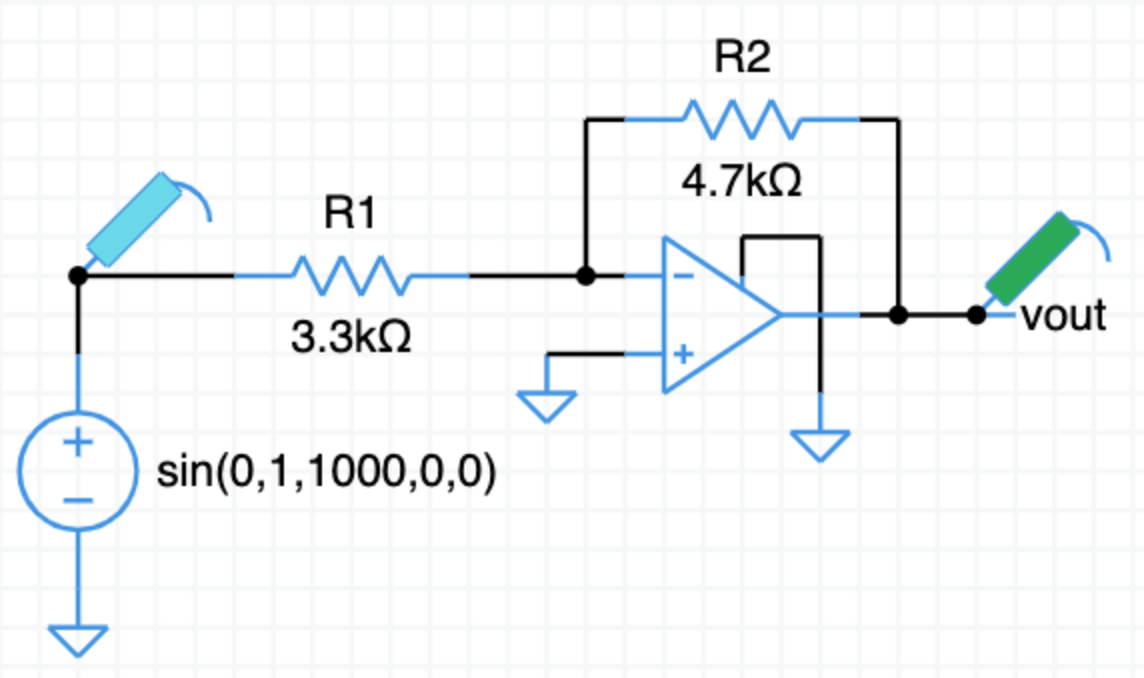What is the expected gain of this amplifier?

From the inverting opamp video, the gain expression for the amplifier is,

$v_o = -\dfrac{\text R2}{\text R1}\,v_{in}$

Fill in the resistor values,

$v_o = -\dfrac{4.7\text k}{3.3\text k}\,v_{in}$

$v_o = -\dfrac{4.7}{3.3}\,v_{in}$

$v_o = -1.4\,v_{in}$

The gain is $-1.4$. The output is inverted with respect to the input and is $1.4$ times larger.

Click on TRAN to run a transient simulation.

Does the simulation match your gain calculation?

Design an amplifier with a gain of $-10$.

Hint: Pick new values for either or both of $\text R1$ and $\text R2$.

Change the values of the resistors in the simulation and run TRAN again.

Verify your new amplifier has the gain you expect.

Starting from scratch with a blank schematic, design and simulate an inverting amplifier with a gain of $-1$.

Circuit sandbox